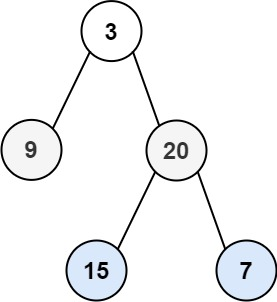## C# || How To Traverse Bottom Up Binary Tree Level Order Using C#The following is a module with functions which demonstrates how to traverse bottom up binary tree level order using C#.

1. Level Order Bottom – Problem Statement

Given the root of a binary tree, return the bottom-up level order traversal of its nodes’ values. (i.e., from left to right, level by level from leaf to root).

Example 1:``` Input: root = [3,9,20,null,null,15,7] Output: [[15,7],[9,20],] ```

Example 2:

``` Input: root =  Output: [] ```

Example 3:

``` Input: root = [] Output: [] ```

2. Level Order Bottom – Solution

The following is a solution which demonstrates how to traverse bottom up binary tree level order.

The idea of this solution is to have a result list which keeps track of the items found on each level. A variable is also used to keep track of the maximum depth levels in the tree. The max depth level is used to insert node values into their appropriate result list slot.

``` 2. Level Order Bottom - Solution C# // ============================================================================ // Author: Kenneth Perkins // Date: Oct 20, 2021 // Taken From: http://programmingnotes.org/ // File: Solution.cs // Description: Determines how to traverse a tree bottom up level order // ============================================================================ /** * Definition for a binary tree node. * public class TreeNode { * public int val; * public TreeNode left; * public TreeNode right; * public TreeNode(int val=0, TreeNode left=null, TreeNode right=null) { * this.val = val; * this.left = left; * this.right = right; * } * } */ public class Solution { private List<IList<int>> result = new List<IList<int>>(); private int maxDepth = 0; public IList<IList<int>> LevelOrderBottom(TreeNode root) { Traverse(root, 0); return result; } private void Traverse(TreeNode node, int depth) { if (node == null) { return; } // Keep track of max depth level if (depth > maxDepth) { maxDepth = depth; } // Determine if new depth level should be added to the result list // Add the new level to the start of the result list if (result.Count == depth) { result.Insert(0, new List<int>()); } // Add the current node value to the corresponding result level index result[maxDepth - depth].Add(node.val); // Keep exploring left and right nodes Traverse(node.left, depth + 1); Traverse(node.right, depth + 1); } }// http://programmingnotes.org/ 1234567891011121314151617181920212223242526272829303132333435363738394041424344454647484950515253 // ============================================================================//    Author: Kenneth Perkins//    Date:   Oct 20, 2021//    Taken From: http://programmingnotes.org///    File:  Solution.cs//    Description: Determines how to traverse a tree bottom up level order// ============================================================================/** * Definition for a binary tree node. * public class TreeNode { *     public int val; *     public TreeNode left; *     public TreeNode right; *     public TreeNode(int val=0, TreeNode left=null, TreeNode right=null) { *         this.val = val; *         this.left = left; *         this.right = right; *     } * } */public class Solution {    private List<IList<int>> result = new List<IList<int>>();    private int maxDepth = 0;     public IList<IList<int>> LevelOrderBottom(TreeNode root) {        Traverse(root, 0);        return result;    }     private void Traverse(TreeNode node, int depth) {        if (node == null) {            return;        }         // Keep track of max depth level        if (depth > maxDepth) {            maxDepth = depth;        }         // Determine if new depth level should be added to the result list        // Add the new level to the start of the result list        if (result.Count == depth) {            result.Insert(0, new List<int>());        }         // Add the current node value to the corresponding result level index        result[maxDepth - depth].Add(node.val);         // Keep exploring left and right nodes        Traverse(node.left, depth + 1);        Traverse(node.right, depth + 1);    }}// http://programmingnotes.org/ ```

QUICK NOTES:
The highlighted lines are sections of interest to look out for.

The code is heavily commented, so no further insight is necessary. If you have any questions, feel free to leave a comment below.

Once compiled, you should get this as your output for the example cases:

``` [[15,7],[9,20],] [] [] ```